# 图像分析：分水岭算法解析

——————————————————————————————

## 1、算法来源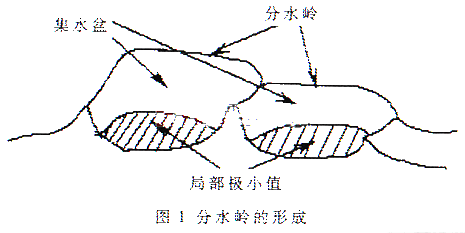## 2、数学描述

Cn(Mi)=C(Mi)&T[n]      (1)  （即是说Cn(Mi)表示一个区域，它既是与Mi为极小值点的一个连通流域同时这个区域的灰度值小于n）

1) D&C[n-1]为空；
2) D&C[n-1]为非空，含有C[n-1]一个连通成分；
3) D&C[n-1]为非空，含有C[n-1]多个连通成分。

## 3、程序实现

f = imread('E:\快盘资料\学习文档\冈萨雷斯数字图像处理 图像和代码\myimage\dipum_images_ch10\f1.tif');
figure,imshow(f);
title('原始图像')

f = double(f);
g = im2bw(f,graythresh(f));
figure,imshow(g)
title('二值图像')
gc = ~g;
figure,imshow(gc)
title('图像的补')
D = bwdist(gc);
figure,imshow(D)
title('')
L = watershed(-D);
w = L == 0;
figure,imshow(w)

g2 = g & ~w;
figure,imshow(g2)
title('最后的分水岭线')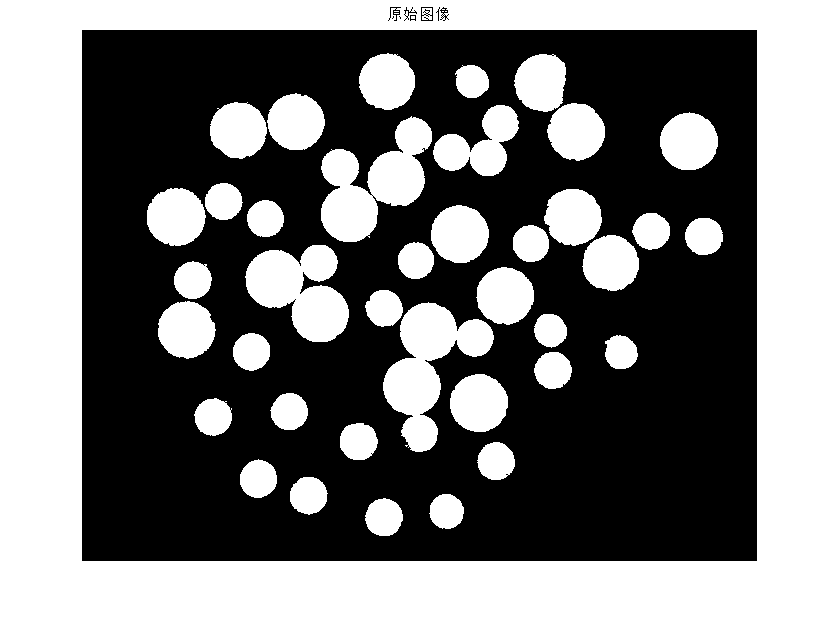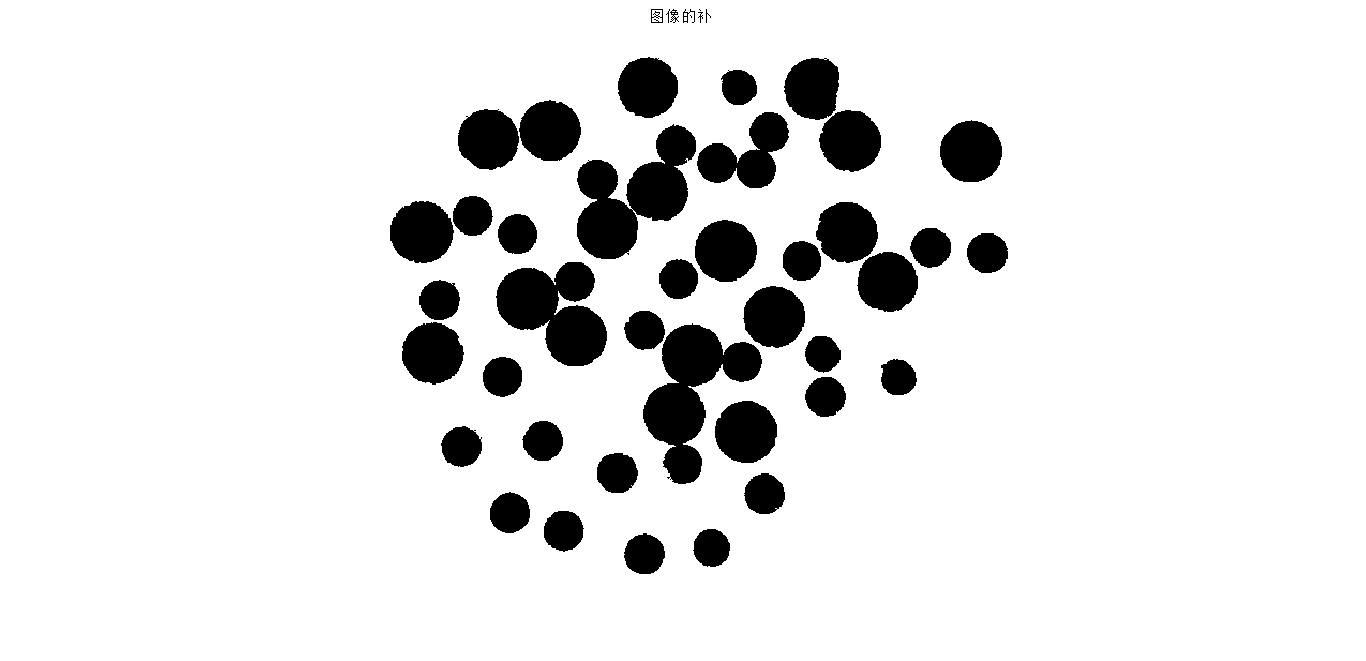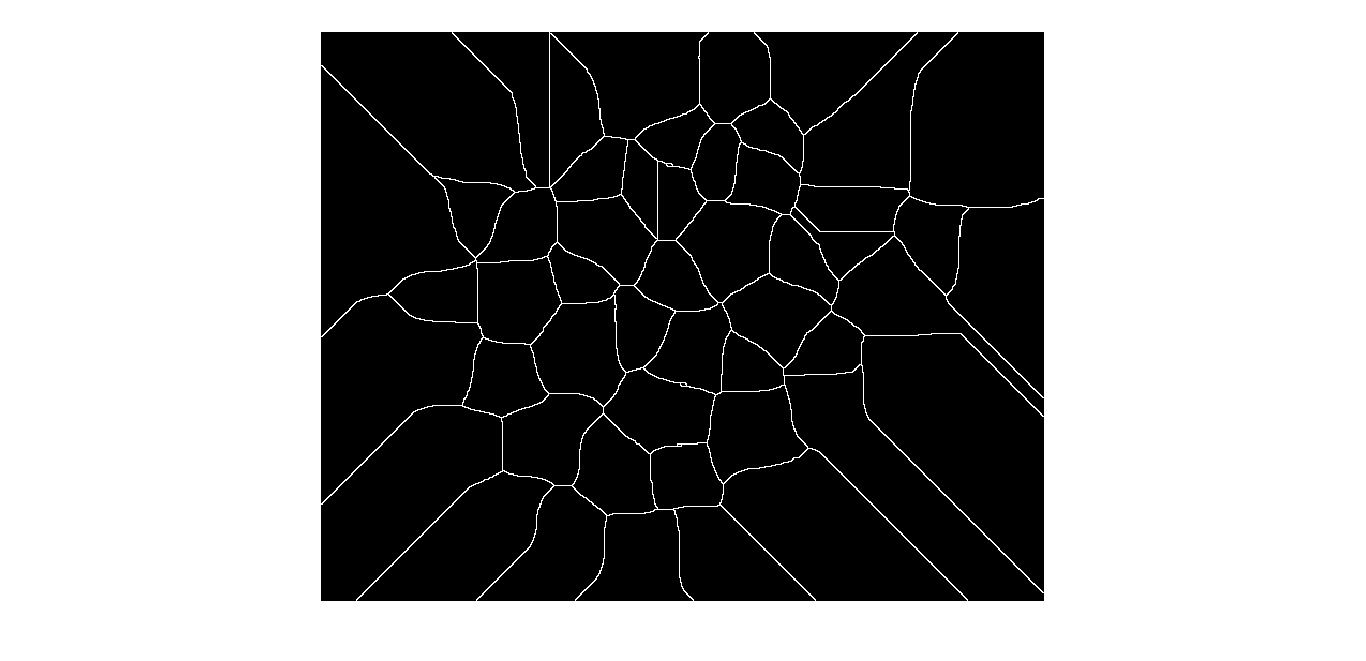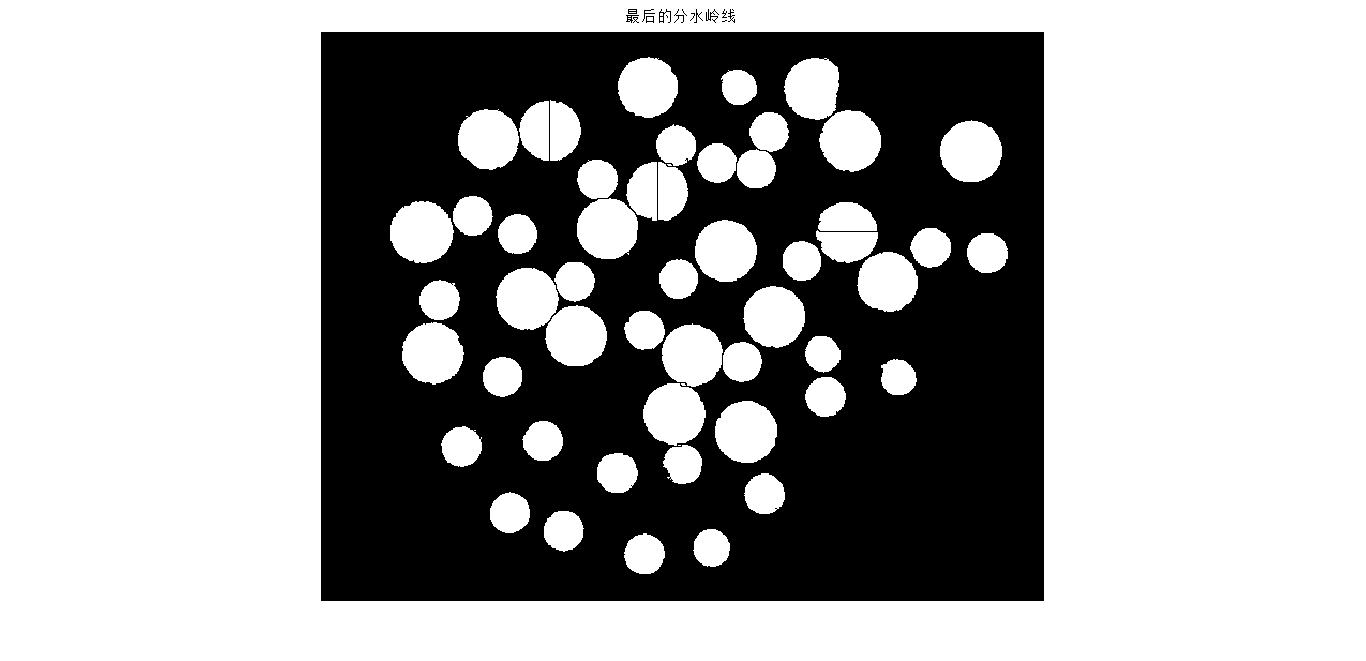%2-D Example
%Make a binary image containing two overlapping circular objects.
center1 = -10;
center2 = -center1;
dist = sqrt(2*(2*center1)^2);
radius = dist/2 * 1.4;
lims = [floor(center1-1.2*radius) ceil(center2+1.2*radius)];
[x,y] = meshgrid(lims(1):lims(2));
bw1 = sqrt((x-center1).^2 + (y-center1).^2) <= radius;
bw2 = sqrt((x-center2).^2 + (y-center2).^2) <= radius;
bw = bw1 | bw2;
figure, imshow(bw,'InitialMagnification','fit'), title('bw')
%Compute the distance transform of the complement of the binary image.
D = bwdist(~bw);
figure, imshow(D,[],'InitialMagnification','fit')
title('Distance transform of ~bw')
%Complement the distance transform, and force pixels that don't belong to the objects to be at -Inf.
D = -D;
D(~bw) = -Inf;
%Compute the watershed transform and display the resulting label matrix as an RGB images.
L = watershed(D);
rgb = label2rgb(L,'jet',[.5 .5 .5]);
figure, imshow(rgb,'InitialMagnification','fit')
title('Watershed transform of D')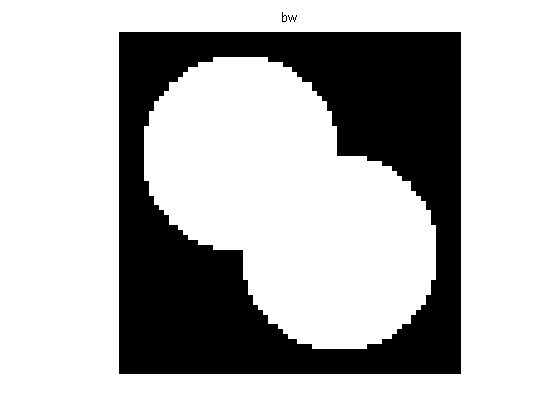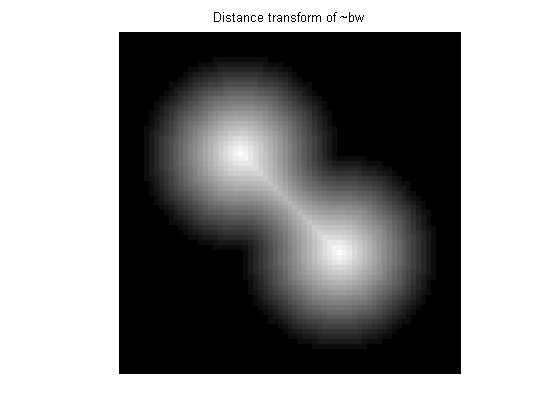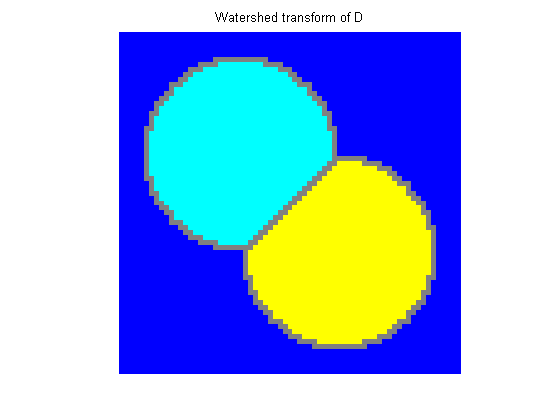参考文献：

   Vincent L, Soille P. Watersheds in digital spaces: an efficient algorithm based on immersion simulations[J]. IEEE transactions on pattern analysis and machine intelligence, 1991, 13(6): 583-598.

   马丽红, 张宇, 邓健平. 基于形态开闭滤波二值标记和纹理特征合并的分水岭算法[J]. 中国图象图形学报, 2003, 1.
   王国权, 周小红, 蔚立磊. 基于分水岭算法的图像分割方法研究 [J]. 计算机仿真, 2009, 26(5): 255-258.
  松卡, 赫拉瓦奇, 博伊尔. 图像处理, 分析与机器视觉[M]. 人民邮电出版社, 2002.